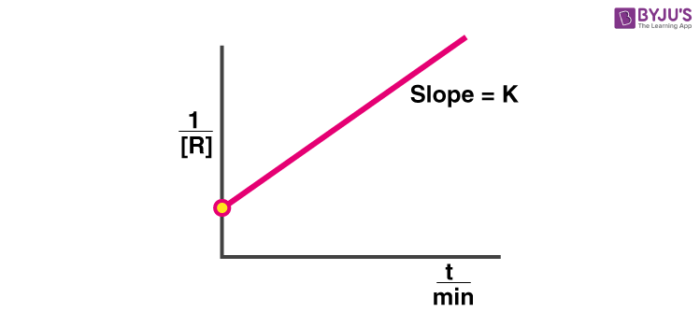# Second Order Reaction

Second order reactions can be defined as chemical reactions wherein the sum of the exponents in the corresponding rate law of the chemical reaction is equal to two. The rate of such a reaction can be written either as r = k[A]2, or as r = k[A][B].

## What is a Second Order Reaction?

From the rate law equations given above, it can be understood that second order reactions are chemical reactions which depend on either the concentrations of two first-order reactants or the concentration of one-second order reactant.

Since second order reactions can be of the two types described above, the rate of these reactions can be generalized as follows:

r = k[A]x[B]y

Where the sum of x and y (which corresponds to the order of the chemical reaction in question) equals two.

### Examples of Second Order Reactions

A few examples of second order reactions are given below:

$$H^{+} + OH^{-} \rightarrow H_{2}O$$ $$C + O_{2} \rightarrow CO + O$$

The two examples given above are the second order reactions depending on the concentration of two separate first order reactants.

$$2NO_{2} \rightarrow 2NO + O_{2}$$ $$2HI \rightarrow I_{2} + H_{2}$$

These reactions involve one second order reactant yielding the product.

## Differential and Integrated Rate Equation for Second Order Reactions

Considering the scenario where one second order reactant forms a given product in a chemical reaction, the differential rate law equation can be written as follows:

$$\frac{-d[R]}{dt} = k[R]^{2}$$

In order to obtain the integrated rate equation, this differential form must be rearranged as follows:

$$\frac{d[R]}{[R]^{2}} = -k dt$$

Now, integrating on both sides in consideration of the change in the concentration of reactant between time 0 and time t, the following equation is attained.

$$\int_{[R]_{0}}^{[R]_{t}} \frac{d[R]}{[R]^{2}} = -k \int_{0}^{t} dt$$

From the power rule of integration, we have:

$$\int \frac{dx}{x^{2}} = -\frac{1}{x} + C$$

Where C is the constant of Integration. Now, using this power rule in the previous equation, the following equation can be attained.

$$\frac{1}{[R]_{t}} – \frac{1}{[R]_{0}} = kt$$

Which is the required integrated rate expression of second order reactions.

## Graph of a Second Order Reaction

Generalizing [R]t as [R] and rearranging the integrated rate law equation of reactions of the second order, the following reaction is obtained.

$$\frac{1}{[R]} = kt + \frac{1}{[R]_{0}}$$

Plotting a straight line (y=mx + c) corresponding to this equation (y = 1/[R] , x = t , m = k , c = 1/[R]0)It can be observed that the slope of the straight line is equal to the value of the rate constant, k.

## Half-Life of Second-Order Reactions

The half-life of a chemical reaction is the time taken for half of the initial amount of reactant to undergo the reaction.

Therefore, while attempting to calculate the half life of a reaction, the following substitutions must be made:

$$[R] = \frac{[R]_{0}}{2}$$

And, $$t = t_{1/2}$$

Now, substituting these values in the integral form of the rate equation of second order reactions, we get:

$$\frac{1}{\frac{[R]_{0}}{2}} – \frac{1}{[R]_{0}} = kt_{1/2}$$

Therefore, the required equation for the half life of second order reactions can be written as follows.

$$t_{1/2} = \frac{1}{k[R]_{0}}$$

This equation for the half life implies that the half life is inversely proportional to the concentration of the reactants.

## Frequently Asked Questions In Exam:

1. What is meant by the second-order reaction?
2. What are the units of k for a second order reaction?
3. What is the rate law for a second-order reaction?
4. What are the units of a second order reaction?
5. Are second order reactions faster than first order?
6. What is 2nd order kinetics?
7. What is the second-order rate law?
8. How do you know if a graph is a first or second order?
9. What is the equation for the half-life of a second-order process?
10. What is the second order integrated rate law?